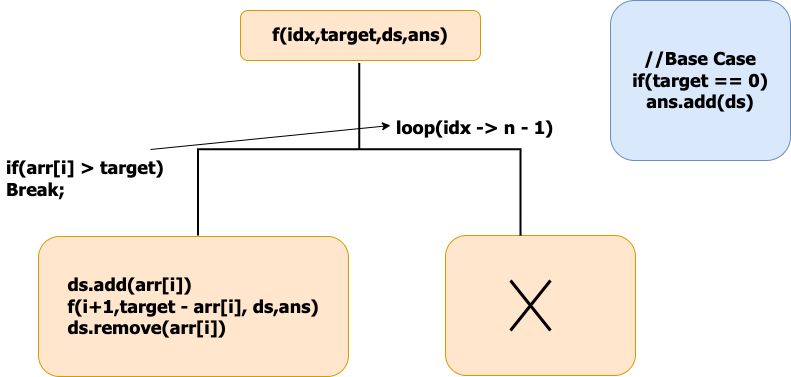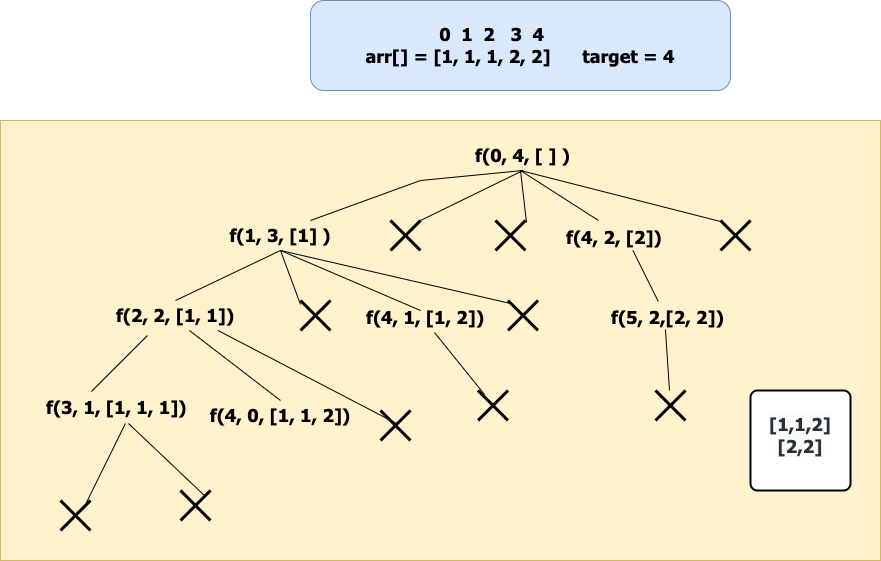# Combination Sum II – Find all unique combinations

In this article we will solve the most asked interview question “Combination Sum II – Find all unique combinations”.

Problem Statement: Given a collection of candidate numbers (candidates) and a target number (target), find all unique combinations in candidates where the candidate numbers sum to target. Each number in candidates may only be used once in the combination.

Note: The solution set must not contain duplicate combinations.

Examples:

```Example 1:

Input: candidates = [10,1,2,7,6,1,5], target = 8

Output:
[
[1,1,6],
[1,2,5],
[1,7],
[2,6]]

Explanation: These are the unique combinations whose sum is equal to target.

Example 2:

Input: candidates = [2,5,2,1,2], target = 5

Output: [[1,2,2],]

Explanation: These are the unique combinations whose sum is equal to target.```

Solution:

Disclaimer: Don’t jump directly to the solution, try it out yourself first.

Solution 1: Using extra space and time complexity

Approach:

Defining the Recursive Function:Before starting the recursive call make sure to sort the elements because the ans should contain the combinations in sorted order and should not be repeated.

Initially, We start with the index 0, At index 0 we have n – 1 way to pick the first element of our subsequence.

Check if the current index value can be added to our ds. If yes add it to the ds and move the index by 1. while moving the index skip the consecutive repeated elements because they will form duplicate sequences.

Reduce the target by arr[i],call the recursive call for f(idx + 1,target – 1,ds,ans) after the call make sure to pop the element from the ds.(By seeing the example recursive You will understand).

if(arr[i] > target) then terminate the recursive call because there is no use to check as the array is sorted in the next recursive call the index will be moving by 1 all the elements to its right will be in increasing order.

Base Condition:

Whenever the target value is zero add the ds to the ans return.

Representation of Recursive call for the example given below:If we observe the recursive call for f(2,2,[1,1]) when it is returning the ds doesn’t include 1 so make sure to remove it from ds after the call.

Code:

## C++ Code

``````#include<bits/stdc++.h>

using namespace std;
void findCombination(int ind, int target, vector < int > & arr, vector < vector < int >> & ans, vector < int > & ds) {
if (target == 0) {
ans.push_back(ds);
return;
}
for (int i = ind; i < arr.size(); i++) {
if (i > ind && arr[i] == arr[i - 1]) continue;
if (arr[i] > target) break;
ds.push_back(arr[i]);
findCombination(i + 1, target - arr[i], arr, ans, ds);
ds.pop_back();
}
}
vector < vector < int >> combinationSum2(vector < int > & candidates, int target) {
sort(candidates.begin(), candidates.end());
vector < vector < int >> ans;
vector < int > ds;
findCombination(0, target, candidates, ans, ds);
return ans;
}
int main() {
vector<int> v{10,1,2,7,6,1,5};
vector < vector < int >> comb = combinationSum2(v, 8);
cout << "[ ";
for (int i = 0; i < comb.size(); i++) {
cout << "[ ";
for (int j = 0; j < comb[i].size(); j++) {
cout << comb[i][j] << " ";
}
cout << "]";
}
cout << " ]";
}``````

Output:

[ [ 1 1 6 ][ 1 2 5 ][ 1 7 ][ 2 6 ] ]

Time Complexity:O(2^n*k)

Reason: Assume if all the elements in the array are unique then the no. of subsequence you will get will be O(2^n). we also add the ds to our ans when we reach the base case that will take “k”//average space for the ds.

Space Complexity:O(k*x)

Reason: if we have x combinations then space will be x*k where k is the average length of the combination.

## Java Code

``````import java.util.*;
class TUF {
static void findCombinations(int ind, int[] arr, int target, List < List < Integer >> ans, List < Integer > ds) {
if (target == 0) {
ans.add(new ArrayList < > (ds));
return;
}

for (int i = ind; i < arr.length; i++) {
if (i > ind && arr[i] == arr[i - 1]) continue;
if (arr[i] > target) break;

findCombinations(i + 1, arr, target - arr[i], ans, ds);
ds.remove(ds.size() - 1);
}
}
public static List < List < Integer >> combinationSum2(int[] candidates, int target) {
List < List < Integer >> ans = new ArrayList < > ();
Arrays.sort(candidates);
findCombinations(0, candidates, target, ans, new ArrayList < > ());
return ans;
}

public static void main(String args[]) {
int v[]={10,1,2,7,6,1,5};
List < List < Integer >> comb = combinationSum2(v, 8);
System.out.println(comb.toString().replace(",", " "));
}
}``````

Output:

[ [ 1 1 6 ][ 1 2 5 ][ 1 7 ][ 2 6 ] ]

Time Complexity:O(2^n*k)

Reason: Assume if all the elements in the array are unique then the no. of subsequence you will get will be O(2^n). we also add the ds to our ans when we reach the base case that will take “k”//average space for the ds.

Space Complexity:O(k*x)

Reason: if we have x combinations then space will be x*k where k is the average length of the combination.

Special thanks to P.C.Bhuvaneshwari for contributing to this article on takeUforward. If you also wish to share your knowledge with the takeUforward fam, please check out this article